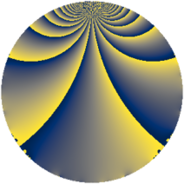# Properties

 Label 2850.2.rLevel $2850$ Weight $2$ Character orbit 2850.r Rep. character $\chi_{2850}(49,\cdot)$ Character field $\Q(\zeta_{6})$ Dimension $120$ Sturm bound $1200$

# Learn more about

## Defining parameters

 Level: $$N$$ $$=$$ $$2850 = 2 \cdot 3 \cdot 5^{2} \cdot 19$$ Weight: $$k$$ $$=$$ $$2$$ Character orbit: $$[\chi]$$ $$=$$ 2850.r (of order $$6$$ and degree $$2$$) Character conductor: $$\operatorname{cond}(\chi)$$ $$=$$ $$95$$ Character field: $$\Q(\zeta_{6})$$ Sturm bound: $$1200$$

## Dimensions

The following table gives the dimensions of various subspaces of $$M_{2}(2850, [\chi])$$.

Total New Old
Modular forms 1248 120 1128
Cusp forms 1152 120 1032
Eisenstein series 96 0 96

## Trace form

 $$120q + 60q^{4} + 60q^{9} + O(q^{10})$$ $$120q + 60q^{4} + 60q^{9} - 32q^{11} - 8q^{14} - 60q^{16} + 8q^{19} + 4q^{21} + 32q^{26} + 16q^{29} + 8q^{31} - 16q^{34} - 60q^{36} - 8q^{39} + 8q^{41} - 16q^{44} - 80q^{46} - 96q^{49} + 8q^{51} - 16q^{56} - 16q^{59} - 12q^{61} - 120q^{64} + 16q^{69} - 80q^{71} - 24q^{74} + 28q^{76} + 4q^{79} - 60q^{81} + 8q^{84} + 16q^{86} + 80q^{89} + 68q^{91} - 16q^{99} + O(q^{100})$$

## Decomposition of $$S_{2}^{\mathrm{new}}(2850, [\chi])$$ into newform subspaces

The newforms in this space have not yet been added to the LMFDB.

## Decomposition of $$S_{2}^{\mathrm{old}}(2850, [\chi])$$ into lower level spaces

$$S_{2}^{\mathrm{old}}(2850, [\chi]) \cong$$ $$S_{2}^{\mathrm{new}}(95, [\chi])$$$$^{\oplus 8}$$$$\oplus$$$$S_{2}^{\mathrm{new}}(190, [\chi])$$$$^{\oplus 4}$$$$\oplus$$$$S_{2}^{\mathrm{new}}(285, [\chi])$$$$^{\oplus 4}$$$$\oplus$$$$S_{2}^{\mathrm{new}}(475, [\chi])$$$$^{\oplus 4}$$$$\oplus$$$$S_{2}^{\mathrm{new}}(570, [\chi])$$$$^{\oplus 2}$$$$\oplus$$$$S_{2}^{\mathrm{new}}(950, [\chi])$$$$^{\oplus 2}$$$$\oplus$$$$S_{2}^{\mathrm{new}}(1425, [\chi])$$$$^{\oplus 2}$$